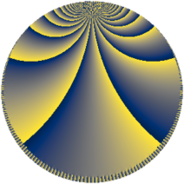# Properties

 Label 384.8.kLevel $384$ Weight $8$ Character orbit 384.k Rep. character $\chi_{384}(95,\cdot)$ Character field $\Q(\zeta_{4})$ Dimension $216$ Sturm bound $512$

# Related objects

## Defining parameters

 Level: $$N$$ $$=$$ $$384 = 2^{7} \cdot 3$$ Weight: $$k$$ $$=$$ $$8$$ Character orbit: $$[\chi]$$ $$=$$ 384.k (of order $$4$$ and degree $$2$$) Character conductor: $$\operatorname{cond}(\chi)$$ $$=$$ $$48$$ Character field: $$\Q(i)$$ Sturm bound: $$512$$

## Dimensions

The following table gives the dimensions of various subspaces of $$M_{8}(384, [\chi])$$.

Total New Old
Modular forms 928 232 696
Cusp forms 864 216 648
Eisenstein series 64 16 48

## Trace form

 $$216 q + O(q^{10})$$ $$216 q + 8 q^{13} + 8752 q^{21} - 8 q^{33} + 8 q^{37} + 312504 q^{45} + 19765016 q^{49} + 4559784 q^{61} - 8744 q^{69} - 8 q^{81} - 15692992 q^{85} + 28990792 q^{93} - 16 q^{97} + O(q^{100})$$

## Decomposition of $$S_{8}^{\mathrm{new}}(384, [\chi])$$ into newform subspaces

The newforms in this space have not yet been added to the LMFDB.

## Decomposition of $$S_{8}^{\mathrm{old}}(384, [\chi])$$ into lower level spaces

$$S_{8}^{\mathrm{old}}(384, [\chi]) \cong$$ $$S_{8}^{\mathrm{new}}(48, [\chi])$$$$^{\oplus 4}$$$$\oplus$$$$S_{8}^{\mathrm{new}}(192, [\chi])$$$$^{\oplus 2}$$